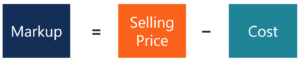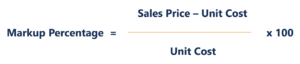# Markup

The difference between the selling price of a good or service and its cost

## What is Markup?

Markup refers to the difference between the selling price of a good or service and its cost. It is expressed as a percentage above the cost. In other words, it is the added price over the total cost of the good or service that provides the seller with a profit.Image: CFI’s Free Financial Analyst Courses.

### Markup Percentage Formula

The formula for calculating markup percentage can be expressed as:For example, if a product costs \$10 and the selling price is \$15, the markup percentage would be (\$15 – \$10) / \$10 = 0.50 x 100 = 50%.

### Example

John is the owner of a company that specializes in the manufacturing of office computers and printers. He recently received a large order from a company for 30 computers and 5 printers. In addition, the company tasked John with installing software into each of the computers.

The cost per computer is \$500 and the cost per printer is \$100. The cost of installing the software to run on all the computers is \$2,000. If John wants to earn a 20% profit for the order, what would be the price he needs to charge?

Step 1: Calculate the total cost of the order (computers + printers + installation of software). \$500 x 30 + \$100 x 5 + \$2,000 = \$17,500 (total cost).

Step 2: Determine the selling price by using the desired percentage of 20%. 20% = (Selling Price – \$17,500) / \$17,500  therefore Selling price must be: \$21,000 (selling price).

Therefore, for John to achieve the desired markup percentage of 20%, John would need to charge the company \$21,000.

### The Importance of Understanding Markup

Understanding markup is very important for a business. For example, establishing a pricing strategy is one of the most important parts of strategic pricing. The markup of a good or service must be enough to offset all business expenses and generate a profit.

### The Difference Between Markup and Gross Margin

A lot of people use the terms markup and gross margin interchangeably. Although both terms are used to help determine profitability, they are different!

Markup is the difference between a product’s selling price and cost as a percentage of the cost. For example, if a product sells for \$125 and costs \$100, the additional price increase is (\$125 – \$100) / \$100) x 100 = 25%.

Gross margin is the difference between a product’s selling price and the cost as a percentage of revenue. For example, if a product sells for \$125 and costs \$100, the gross margin is (\$125 – \$100) / \$125 = 0.2(20%) = 20%.

Recall the example above. The gross margin would be (\$21,000 – \$17,500) / \$21,000 = 0.1667 = 16.67%. While the markup was 20%

Intuitively, the markup is always larger, as compared to the gross margin, as shown in the table below. (As long as you charge more than what the product costs.)

MarkupMargin
11%10%
25%20%
66.7%40%
100%50%

### Markups in Different Industries

Markup percentage varies greatly depending on the industry. In some industries, the increase is a tiny percentage (5%-10%) of the total cost of the product or service, while other industries are able to mark up their products or services by an extraordinarily high amount. Therefore, there is no “normal” markup percentage that applies to all products, although there may be an average for a particular industry. Learn more about industry analysis in CFI’s Financial Analyst Training Program.

Thank you for reading this CFI guide to Markup. At CFI, our mission is to help anyone in the world become a first-class financial analyst and master the art of financial modeling and valuation.  To keep learning and advancing your career, these additional CFI resources will be helpful:

• Net Income
• Operating Margin
• Sales Revenue
• Projecting Income Statement Line Items